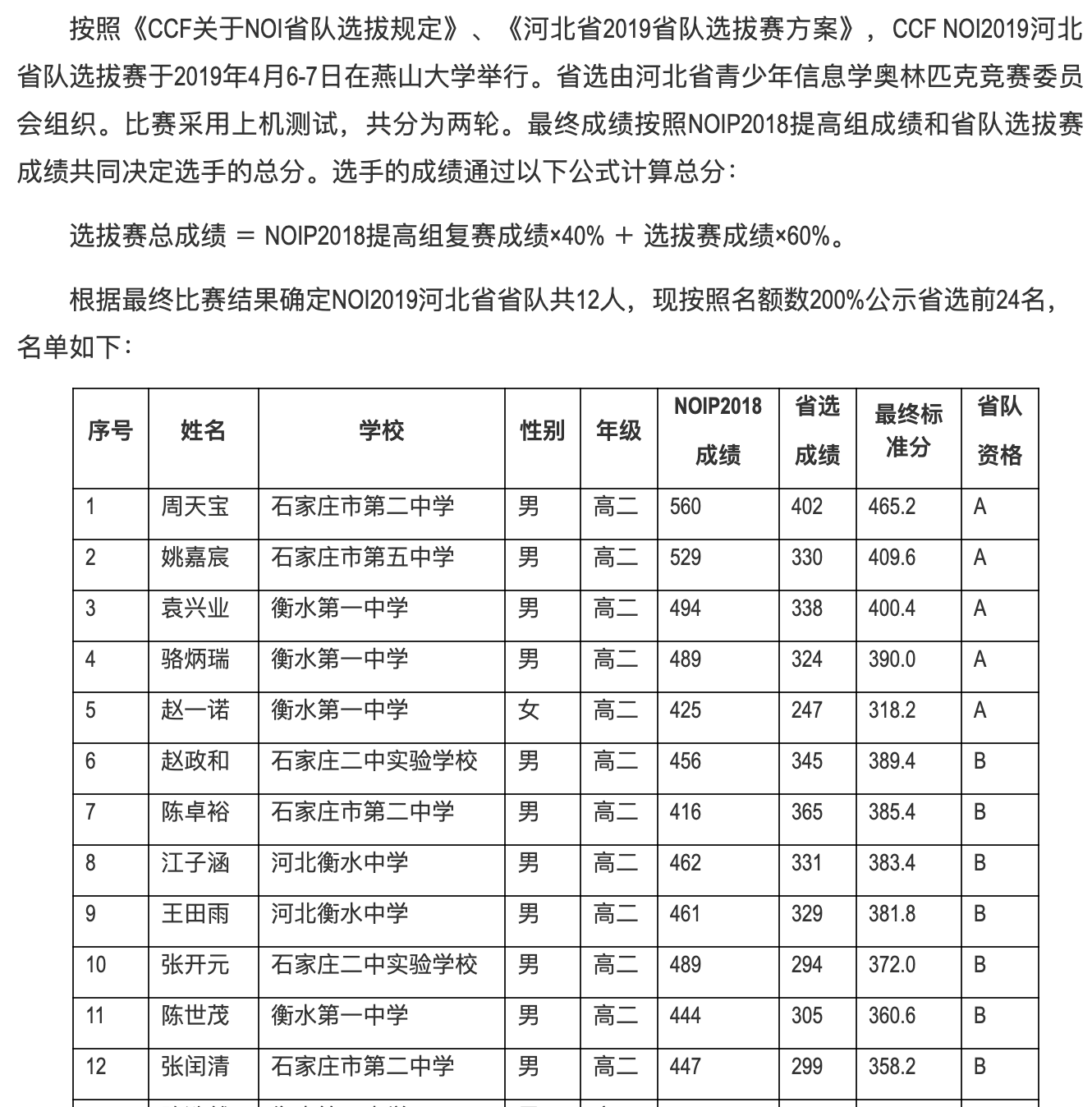# HEOI2019 游记

$\mathfrak{the} \,\, \mathfrak{wind} \,\, \mathfrak{and} \,\, \mathfrak{rain} \,\, \mathfrak{is} \,\, \mathfrak{in} \,\, \mathfrak{my} \,\, \mathfrak{way} \,\, \mathfrak{and} \,\, \mathfrak{never} \,\, \mathfrak{going} \,\, \mathfrak{away}$

# day -???

noip 考挂了，校队垫底，上一名虐我 $50pts+$……

# day -??

• 笛卡尔树

• 解微分方程

• 映射 $fwt$

• 一言不合就三分

• 通过加法等式来实现 $O(n)$ 变 $O(\sqrt n)$

• 二分图匹配解决树的子连通块同构判定

• 大分类讨论

• 数据分治 $fft$

• 区间乘矩阵的幂的预处理少 $O(\log T)$

• 计数的多次幂转化为点对贡献，排列计数转化为从小到大插入

# day 2

$$416 \times 0.4 + (197 + 168) \times 0.6 = 385.4$$

（由于第一天做题顺序，与 $+5pts$ 惨惨离手了……）

# day 3

• $D1T1$：$n \le 10^3$ 的 $O(n^2 \log n + k)$，$60pts$
• $D1T2$：$100pts$
• $D1T3$：一些签到分，共 $37pts$
• $D2T1$：$m \le 100$ 的 $O(cm^3)$，共 $40pts$
• $D2T2$：$100pts$
• $D2T3$：暴力枚举子集，然后求合法点数，然后跑 $fwt$，特判 $n=L,k=1$，有 $28pts$• 微信扫一扫